# Question about fluid flowing into branching pipes

• leifh
Read moreIn summary, the conversation discusses the relationship between pipe or duct sizing and pressure and flow rate in a system, as well as the calculations involved in determining these values. The system is described as a series of non-linear equations that can be solved using linearization and iteration. The main factors affecting the system are the Reynolds Number, length, and diameter of the pipes or ducts. f

#### leifh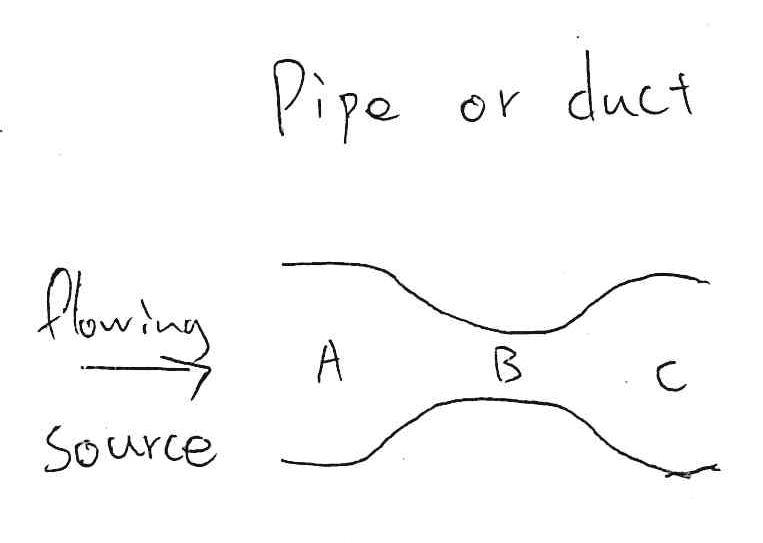For above pipe or duct flowing (where point A and C are higher diameter than B), same volume flow for point A,B & C.
Velocity B > Velocity A & C ; Pressure energy A > Pressure energy B ; friction pressure B > friction pressure A.
Question one: When pipe or duct sizing to be reducing even re-increasing afterward, the pressure will always drop down and never increase again in the same system without power addition in midway of system?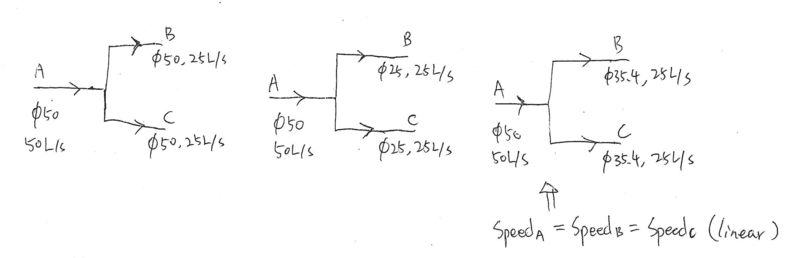As I known, the flow rate will be averagely divided to the same size of pipe or duct.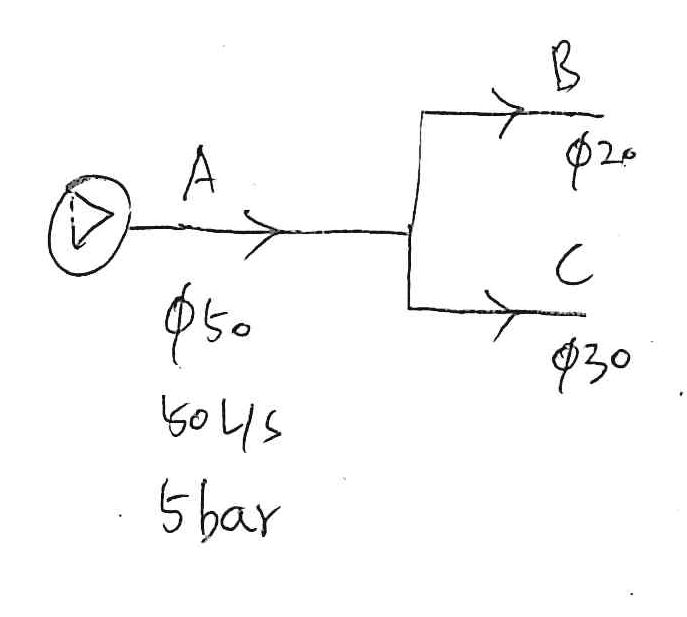Question two: Could we calculate the volume flow rate & pressure for point B and C which only know size?
(assume the source provides steady and constant power, same length of branch pipe or duct, elbow and fluid: water, constant temp. etc)

1. No. Energy is conserved (not pressure). Energy is a function of pressure and velocity.
2. Yes.

Given some information for the length of piping, and components ( like Elbows, Tee's, Reducers etc..), and the conditions at A, namely pressure, flow rate, and the outlet conditions, then yeah, we can specify pressures at various locations in the system, and flow in each branch.

If the flow is steady (not varying in time) and the fluid is "virtually incompressible" (like water) then you can apply the following result between any two points in the system:

$$\frac{P_1}{ \gamma} + z_1 + \frac{V_1^2}{2g} + h_p = \frac{P_2}{\gamma} + z_2 + \frac{V_2^2}{2g} + h_t + \sum_{1 \to 2} h_L$$

Each of the terms represents a form of "head", which basically an energy per unit mass of an element of flowing fluid.

## \frac{P}{\gamma} ## is a pressure head
## z ## is an elevation head
## \frac{V_1^2}{2g} ## is a kinetic energy head
## h_p ## is a pump head (external energy input)
## h_t ## is a turbine head (external energy withdrawal)

## \sum_{1 \to 2} h_L ## represents a thermal head that is created by viscous interaction ( friction) between the fluid and the pipe walls. Its always positive from the Second Law of Thermodynamics, and it is proportional to the kinetic energy head.

For the system you have shown this is going to amount to solving a system of non-linear equations resembling something like the following:

$$\begin{array} \, \frac{P_A}{ \gamma } + \frac{V_A^2}{2g} = \frac{P_{A'} }{\gamma} + \frac{V_{A'}^2}{2g} + k_A ( Re, L , D) \frac{V_{A'}^2}{2g} \\ \frac{P_{A'} }{\gamma} + \frac{V_{A'}^2}{2g} = \frac{P_B}{\gamma} + \frac{V_B^2}{2g} + k_B( Re, L , D) \frac{V_B^2}{2g} \\ \frac{P_{A'} }{\gamma} + \frac{V_{A'}^2}{2g} = \frac{V_C^2}{2g} + k_C( Re, L , D) \frac{V_C^2}{2g} \\ Q_A = Q_B + Q_C \end{array}$$

The ##k( Re, L , D)## means it is a function of the Reynolds Number ( which has other dependencies: flow velocity, diameter, kinematic viscosity ), Length ## L ## and Diameter ## D## of pipe.

The system above is usually tackled by linearization and iteration until a desired accuracy for flow rates is achieved.

••Delta2 and leifh
Given some information for the length of piping, and components ( like Elbows, Tee's, Reducers etc..), and the conditions at A, namely pressure, flow rate, and the outlet conditions, then yeah, we can specify pressures at various locations in the system, and flow in each branch.

If the flow is steady (not varying in time) and the fluid is "virtually incompressible" (like water) then you can apply the following result between any two points in the system:

$$\frac{P_1}{ \gamma} + z_1 + \frac{V_1^2}{2g} + h_p = \frac{P_2}{\gamma} + z_2 + \frac{V_2^2}{2g} + h_t + \sum_{1 \to 2} h_L$$

Each of the terms represents a form of "head", which basically an energy per unit mass of an element of flowing fluid.

## \frac{P}{\gamma} ## is a pressure head
## z ## is an elevation head
## \frac{V_1^2}{2g} ## is a kinetic energy head
## h_p ## is a pump head (external energy input)
## h_t ## is a turbine head (external energy withdrawal)

## \sum_{1 \to 2} h_L ## represents a thermal head that is created by viscous interaction ( friction) between the fluid and the pipe walls. Its always positive from the Second Law of Thermodynamics, and it is proportional to the kinetic energy head.

For the system you have shown this is going to amount to solving a system of non-linear equations resembling something like the following:

$$\begin{array} \, \frac{P_A}{ \gamma } + \frac{V_A^2}{2g} = \frac{P_{A'} }{\gamma} + \frac{V_{A'}^2}{2g} + k_A ( Re, L , D) \frac{V_{A'}^2}{2g} \\ \frac{P_{A'} }{\gamma} + \frac{V_{A'}^2}{2g} = \frac{P_B}{\gamma} + \frac{V_B^2}{2g} + k_B( Re, L , D) \frac{V_B^2}{2g} \\ \frac{P_{A'} }{\gamma} + \frac{V_{A'}^2}{2g} = \frac{V_C^2}{2g} + k_C( Re, L , D) \frac{V_C^2}{2g} \\ Q_A = Q_B + Q_C \end{array}$$

The ##k( Re, L , D)## means it is a function of the Reynolds Number ( which has other dependencies: flow velocity, diameter, kinematic viscosity ), Length ## L ## and Diameter ## D## of pipe.

The system above is usually tackled by linearization and iteration until a desired accuracy for flow rates is achieved.
Thank you for your help. erobz :) Really appreciated.
$$\begin{array} \, \frac{P_A}{ \gamma } + \frac{V_A^2}{2g} = \frac{P_{A'} }{\gamma} + \frac{V_{A'}^2}{2g} + k_A ( Re, L , D) \frac{V_{A'}^2}{2g} \\ \frac{P_{A'} }{\gamma} + \frac{V_{A'}^2}{2g} = \frac{P_B}{\gamma} + \frac{V_B^2}{2g} + k_B( Re, L , D) \frac{V_B^2}{2g} \\ \frac{P_{A'} }{\gamma} + \frac{V_{A'}^2}{2g} = \frac{V_C^2}{2g} + k_C( Re, L , D) \frac{V_C^2}{2g} \\ Q_A = Q_B + Q_C \end{array}$$

Would you mind helping to solve the above question two by some steps? As I am not much sure to apply for the equation properly... (you could take assumption for unknown values) Thank you so much!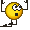Well for starting we are going to need to know the following information:
1. Is the fluid "incompressible" like water
2. What is the fluid (does it have a known viscosity )?
3. Pipe lengths in all sections
4. Discharge pressures at the end of sections B and C
5. Are there specific fittings that we need to know about in a given section of the pipe, elbows, tees, reducers, valves, etc...

Well for starting we are going to need to know the following information:
1. Is the fluid "incompressible" like water
2. What is the fluid (does it have a known viscosity )?
3. Pipe lengths in all sections
4. Discharge pressures at the end of sections B and C
5. Are there specific fittings that we need to know about in a given section of the pipe, elbows, tees, reducers, valves, etc...1. It is water
2. take 20oC temp. Dynamic viscosity of water (in mPa.s) is 1.
3. 10meters for 50mm dia. iron pipe(take same pipe roughness) ; 10m for 20mm dia. iron pipe ; 10m for 30mm dia. iron pipe. they averagely branching to same elbow and not other fitting or valves.
4. assume the water keep flowing and don't know the afterward piping structure and branching, could we know the pressure at point B and C? Or, end of sections B and C is to air (1 atm)
5. a tee 50mm to 20mm/30mm pipe fitting, same elbow and not other fitting or valves

4. assume the water keep flowing and don't know the afterward piping structure and branching, could we know the pressure at point B and C?
Without out a pressure gauge at the desired location, No. The flowrate, and pressure will depend on what is downstream in the piping system in each branch.

Or, end of sections B and C is to air (1 atm)
This criterion can be solved, and is a good first system to get into.

Are there any elevation changes in the pipes relative to the main supply line ( the one bringing in 50 L/s)?

Without out a pressure gauge at the desired location, No. The flowrate, and pressure will depend on what is downstream in the piping system in each branch.

This criterion can be solved, and is a good first system to get into.

Are there any elevation changes in the pipes relative to the main supply line ( the one bringing in 50 L/s)?
no elevation changes, thanks.

Fair warning: This is going to be a little bit of work. However, for this example since you've given us the Flow Rate, it won't actually be terribly difficult. We are not going to get into the iteration of a linearized system, but it will still be instructive.

Alright, can you set up the equation between the inlet of branch A and the Tee junction using the relationship?

You will assume the flow is turbulent and thus "approximately" the velocity is uniformly distributed over the pipe cross sectional area.

Hence:

$$Q_a = V_a A_a$$

*Remember the equation is applicable between any two points ( sections ) along the length of the pipe. For this demonstration, we are going to assume that the head loss coefficient is a constant. So for branch A:

$$k(Re,L,D) = k_a$$

The goal here will be to determine the pressure at the "T" junction.

I forgot to mention the pressures here are gauge pressures, so ##P_{atm} = 0 \, \rm{guage}##

Last edited:
•leifh
Fair warning: This is going to be a little bit of work. However, for this example since you've given us the Flow Rate, it won't actually be terribly difficult. We are not going to get into the iteration of a linearized system, but it will still be instructive.

Alright, can you set up the equation between the inlet of branch A and the Tee junction using the relationship?

You will assume the flow is turbulent and thus "approximately" the velocity is uniformly distributed over the pipe cross sectional area.

Hence:

$$Q_a = V_a A_a$$

*Remember the equation is applicable between any two points ( sections ) along the length of the pipe. For this demonstration, we are going to assume that the head loss coefficient is a constant. So for branch A:

$$k(Re,L,D) = k_a$$

The goal here will be to determine the pressure at the "T" junction.

I forgot to mention the pressures here are gauge pressures, so ##P_{atm} = 0 \, \rm{guage}##
I will set the equation like this: right?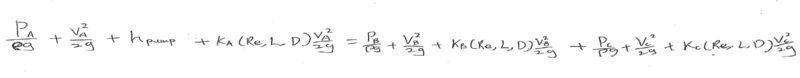How to put values on the below equation? What is K value? (I don't know how to transfer to head form) Is it size of pipe and viscosity relating to kinetic energy head so that K x v2/2g?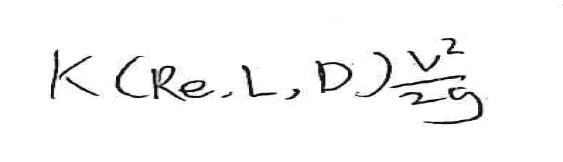Not like that. The equation describes the flow properties between any two points. You have three points considered in what you have shown above.

You have to do this in steps. Image you are a water molecule: Jump into the flow at A, where do you go first before a choice must be made on the remainder of your journey?

We will worry about values later.

Last edited:
$$\frac{P_1}{ \gamma} + z_1 + \frac{V_1^2}{2g} + h_p = \frac{P_2}{\gamma} + z_2 + \frac{V_2^2}{2g} + h_t + \sum_{1 \to 2} h_L$$

I got some applied fluid mechanics tutorials from internet. problem solved. Thanks.

•erobz